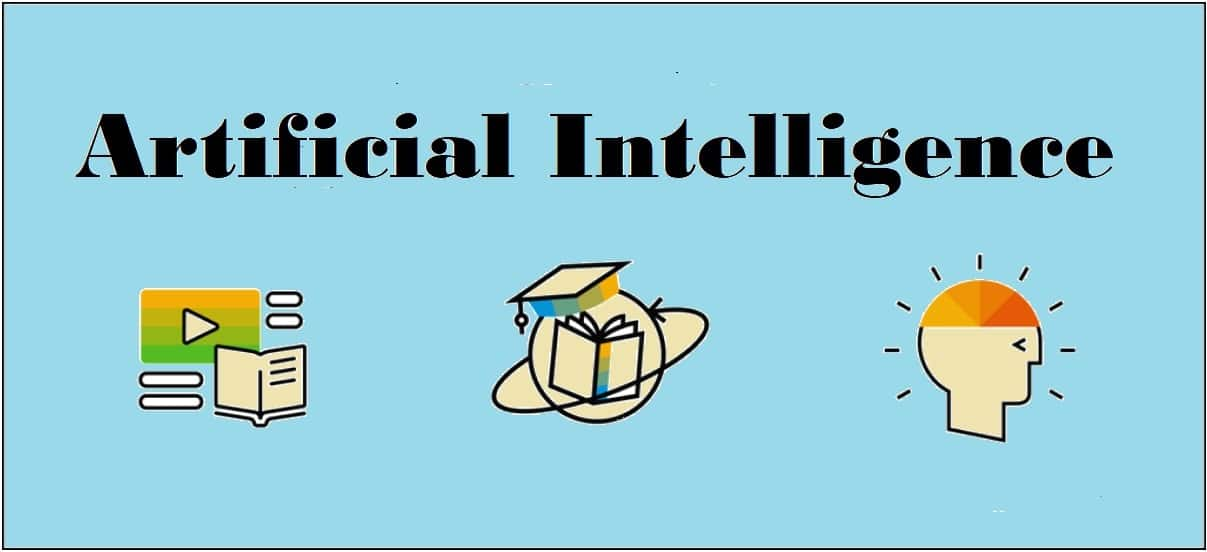## Deep Learning

Deep learning emerged from a decade’s explosive computational growth as a serious contender in the field. Thus, deep learning is a particular kind of machine learning whose algorithms are inspired by the structure and function of human brain.

### Machine Learning vs Deep Learning

Deep learning is the most powerful machine learning technique these days. It is so powerful because they learn the best way to represent the problem while learning how to solve the problem. A comparison of Deep learning and Machine learning is given below −

### Data Dependency

The first point of difference is based upon the performance of DL and ML when the scale of data increases. When the data is large, deep learning algorithms perform very well.

### Machine Dependency

Deep learning algorithms need high-end machines to work perfectly. On the other hand, machine learning algorithms can work on low-end machines too.

### Feature Extraction

Deep learning algorithms can extract high level features and try to learn from the same too. On the other hand, an expert is required to identify most of the features extracted by machine learning.

### Time of Execution

Execution time depends upon the numerous parameters used in an algorithm. Deep learning has more parameters than machine learning algorithms. Hence, the execution time of DL algorithms, specially the training time, is much more than ML algorithms. But the testing time of DL algorithms is less than ML algorithms.

### Approach to Problem Solving

Deep learning solves the problem end-to-end while machine learning uses the traditional way of solving the problem i.e. by breaking down it into parts.

[wpsbx_html_block id=1891]

### Convolutional Neural Network (CNN)

Convolutional neural networks are the same as ordinary neural networks because they are also made up of neurons that have learnable weights and biases. Ordinary neural networks ignore the structure of input data and all the data is converted into 1-D array before feeding it into the network. This process suits the regular data, however if the data contains images, the process may be cumbersome.

CNN solves this problem easily. It takes the 2D structure of the images into account when they process them, which allows them to extract the properties specific to images. In this way, the main goal of CNNs is to go from the raw image data in the input layer to the correct class in the output layer. The only difference between an ordinary NNs and CNNs is in the treatment of input data and in the type of layers.

### Architecture Overview of CNNs

Architecturally, the ordinary neural networks receive an input and transform it through a series of hidden layer. Every layer is connected to the other layer with the help of neurons. The main disadvantage of ordinary neural networks is that they do not scale well to full images.

The architecture of CNNs have neurons arranged in 3 dimensions called width, height and depth. Each neuron in the current layer is connected to a small patch of the output from the previous layer. It is similar to overlaying a 𝑵×𝑵 filter on the input image. It uses M filters to be sure about getting all the details. These M filters are feature extractors which extract features like edges, corners, etc.

### Layers used to construct CNNs

Following layers are used to construct CNNs −

• Input Layer − It takes the raw image data as it is.
• Convolutional Layer − This layer is the core building block of CNNs that does most of the computations. This layer computes the convolutions between the neurons and the various patches in the input.
• Rectified Linear Unit Layer − It applies an activation function to the output of the previous layer. It adds non-linearity to the network so that it can generalize well to any type of function.
• Pooling Layer − Pooling helps us to keep only the important parts as we progress in the network. Pooling layer operates independently on every depth slice of the input and resizes it spatially. It uses the MAX function.
• Fully Connected layer/Output layer − This layer computes the output scores in the last layer. The resulting output is of the size 𝟏×𝟏×𝑳 , where L is the number training dataset classes.

### Installing Useful Python Packages

You can use Keras, which is an high level neural networks API, written in Python and capable of running on top of TensorFlow, CNTK or Theno. It is compatible with Python 2.7-3.6. You can learn more about it from https://keras.io/.

Use the following commands to install keras −

```pip install keras
```

On conda environment, you can use the following command −

```conda install –c conda-forge keras
```

### Building Linear Regressor using ANN

In this section, you will learn how to build a linear regressor using artificial neural networks. You can use KerasRegressor to achieve this. In this example, we are using the Boston house price dataset with 13 numerical for properties in Boston. The Python code for the same is shown here −

Import all the required packages as shown −

```import numpy
import pandas
from keras.models import Sequential
from keras.layers import Dense
from keras.wrappers.scikit_learn import KerasRegressor
from sklearn.model_selection import cross_val_score
from sklearn.model_selection import KFold```

Now, load our dataset which is saved in local directory.

```dataframe = pandas.read_csv("/Usrrs/admin/data.csv", delim_whitespace = True, header = None)
dataset = dataframe.values```

Now, divide the data into input and output variables i.e. X and Y −

```X = dataset[:,0:13]
Y = dataset[:,13]```

Since we use baseline neural networks, define the model −

`def baseline_model():`

Now, create the model as follows −

```model_regressor = Sequential()
model_regressor.add(Dense(13, input_dim = 13, kernel_initializer = 'normal',
activation = 'relu'))

Next, compile the model −

```model_regressor.compile(loss='mean_squared_error', optimizer='adam')
return model_regressor```

Now, fix the random seed for reproducibility as follows −

```seed = 7
numpy.random.seed(seed)```

The Keras wrapper object for use in scikit-learn as a regression estimator is called KerasRegressor. In this section, we shall evaluate this model with standardize data set.

```estimator = KerasRegressor(build_fn = baseline_model, nb_epoch = 100, batch_size = 5, verbose = 0)
kfold = KFold(n_splits = 10, random_state = seed)
baseline_result = cross_val_score(estimator, X, Y, cv = kfold)
print("Baseline: %.2f (%.2f) MSE" % (Baseline_result.mean(),Baseline_result.std()))```

The output of the code shown above would be the estimate of the model’s performance on the problem for unseen data. It will be the mean squared error, including the average and standard deviation across all 10 folds of the cross validation evaluation.

### Image Classifier: An Application of Deep Learning

Convolutional Neural Networks (CNNs) solve an image classification problem, that is to which class the input image belongs to. You can use Keras deep learning library. Note that we are using the training and testing data set of images of cats and dogs from following link https://www.kaggle.com/c/dogs-vs-cats/data.

Import the important keras libraries and packages as shown −

The following package called sequential will initialize the neural networks as sequential network.

`from keras.models import Sequential`

The following package called Conv2D is used to perform the convolution operation, the first step of CNN.

`from keras.layers import Conv2D`

The following package called MaxPoling2D is used to perform the pooling operation, the second step of CNN.

`from keras.layers import MaxPooling2D`

The following package called Flatten is the process of converting all the resultant 2D arrays into a single long continuous linear vector.

`from keras.layers import Flatten`

The following package called Dense is used to perform the full connection of the neural network, the fourth step of CNN.

`from keras.layers import Dense`

Now, create an object of the sequential class.

`S_classifier = Sequential()`

Now, next step is coding the convolution part.

`S_classifier.add(Conv2D(32, (3, 3), input_shape = (64, 64, 3), activation = 'relu'))`

Here relu is the rectifier function.

Now, the next step of CNN is the pooling operation on the resultant feature maps after convolution part.

`S-classifier.add(MaxPooling2D(pool_size = (2, 2)))`

Now, convert all the pooled images into a continuous vector by using flattering −

`S_classifier.add(Flatten())`

Next, create a fully connected layer.

`S_classifier.add(Dense(units = 128, activation = 'relu'))`

Here, 128 is the number of hidden units. It is a common practice to define the number of hidden units as the power of 2.

Now, initialize the output layer as follows −

`S_classifier.add(Dense(units = 1, activation = 'sigmoid'))`

Now, compile the CNN, we have built −

`S_classifier.compile(optimizer = 'adam', loss = 'binary_crossentropy', metrics = ['accuracy'])`

Here optimizer parameter is to choose the stochastic gradient descent algorithm, loss parameter is to choose the loss function and metrics parameter is to choose the performance metric.

Now, perform image augmentations and then fit the images to the neural networks −

```train_datagen = ImageDataGenerator(rescale = 1./255,shear_range = 0.2,
zoom_range = 0.2,
horizontal_flip = True)
test_datagen = ImageDataGenerator(rescale = 1./255)

training_set =
(64, 64),batch_size = 32,class_mode = 'binary')

test_set =
test_datagen.flow_from_directory('test_set',target_size =
(64, 64),batch_size = 32,class_mode = 'binary')```

Now, fit the data to the model we have created −

```classifier.fit_generator(training_set,steps_per_epoch = 8000,epochs =
25,validation_data = test_set,validation_steps = 2000)```

Here steps_per_epoch have the number of training images.

Now as the model has been trained, we can use it for prediction as follows −

```from keras.preprocessing import image

target_size = (64, 64))

test_image = image.img_to_array(test_image)

test_image = np.expand_dims(test_image, axis = 0)

result = classifier.predict(test_image)

training_set.class_indices

if result == 1:
prediction = 'dog'

else:
prediction = 'cat'```## Speech Recognition

Speech is the most basic means of adult human communication. The basic goal of speech processing is to provide an interaction between a human and a machine.

Speech processing system has mainly three tasks −

• First, speech recognition that allows the machine to catch the words, phrases and sentences we speak
• Second, natural language processing to allow the machine to understand what we speak, and
• Third, speech synthesis to allow the machine to speak.

This chapter focuses on speech recognition, the process of understanding the words that are spoken by human beings. Remember that the speech signals are captured with the help of a microphone and then it has to be understood by the system.

### Building a Speech Recognizer

Speech Recognition or Automatic Speech Recognition (ASR) is the center of attention for AI projects like robotics. Without ASR, it is not possible to imagine a cognitive robot interacting with a human. However, it is not quite easy to build a speech recognizer.

### Difficulties in developing a speech recognition system

Developing a high quality speech recognition system is really a difficult problem. The difficulty of speech recognition technology can be broadly characterized along a number of dimensions as discussed below −

• Size of the vocabulary − Size of the vocabulary impacts the ease of developing an ASR. Consider the following sizes of vocabulary for a better understanding.
• A small size vocabulary consists of 2-100 words, for example, as in a voice-menu system
• A medium size vocabulary consists of several 100s to 1,000s of words, for example, as in a database-retrieval task
• A large size vocabulary consists of several 10,000s of words, as in a general dictation task.

Note that, the larger the size of vocabulary, the harder it is to perform recognition.

• Channel characteristics − Channel quality is also an important dimension. For example, human speech contains high bandwidth with full frequency range, while a telephone speech consists of low bandwidth with limited frequency range. Note that it is harder in the latter.
• Speaking mode − Ease of developing an ASR also depends on the speaking mode, that is whether the speech is in isolated word mode, or connected word mode, or in a continuous speech mode. Note that a continuous speech is harder to recognize.
• Speaking style − A read speech may be in a formal style, or spontaneous and conversational with casual style. The latter is harder to recognize.
• Speaker dependency − Speech can be speaker dependent, speaker adaptive, or speaker independent. A speaker independent is the hardest to build.
• Type of noise − Noise is another factor to consider while developing an ASR. Signal to noise ratio may be in various ranges, depending on the acoustic environment that observes less versus more background noise −
• If the signal to noise ratio is greater than 30dB, it is considered as high range
• If the signal to noise ratio lies between 30dB to 10db, it is considered as medium SNR
• If the signal to noise ratio is lesser than 10dB, it is considered as low range

For example, the type of background noise such as stationary, non-human noise, background speech and crosstalk by other speakers also contributes to the difficulty of the problem.

• Microphone characteristics − The quality of microphone may be good, average, or below average. Also, the distance between mouth and micro-phone can vary. These factors also should be considered for recognition systems.

Despite these difficulties, researchers worked a lot on various aspects of speech such as understanding the speech signal, the speaker, and identifying the accents.

You will have to follow the steps given below to build a speech recognizer:

### Visualizing Audio Signals:

Reading from a File and Working on it. This is the first step in building speech recognition system as it gives an understanding of how an audio signal is structured. Some common steps that can be followed to work with audio signals are as follows −

### Recording

When you have to read the audio signal from a file, then record it using a microphone, at first.

### Sampling

When recording with microphone, the signals are stored in a digitized form. But to work upon it, the machine needs them in the discrete numeric form. Hence, we should perform sampling at a certain frequency and convert the signal into the discrete numerical form. Choosing the high frequency for sampling implies that when humans listen to the signal, they feel it as a continuous audio signal.

### Example

The following example shows a stepwise approach to analyze an audio signal, using Python, which is stored in a file. The frequency of this audio signal is 44,100 HZ.

Import the necessary packages as shown here −

```import numpy as np
import matplotlib.pyplot as plt
from scipy.io import wavfile```

Now, read the stored audio file. It will return two values: the sampling frequency and the audio signal. Provide the path of the audio file where it is stored, as shown here −

`frequency_sampling, audio_signal = wavfile.read("/Users/admin/audio_file.wav")`

Display the parameters like sampling frequency of the audio signal, data type of signal and its duration, using the commands shown −

```print('\nSignal shape:', audio_signal.shape)
print('Signal Datatype:', audio_signal.dtype)
print('Signal duration:', round(audio_signal.shape /
float(frequency_sampling), 2), 'seconds')```

This step involves normalizing the signal as shown below −

`audio_signal = audio_signal / np.power(2, 15)`

In this step, we are extracting the first 100 values from this signal to visualize. Use the following commands for this purpose −

```audio_signal = audio_signal [:100]
time_axis = 1000 * np.arange(0, len(signal), 1) / float(frequency_sampling)
```

Now, visualize the signal using the commands given below −

```plt.plot(time_axis, signal, color='blue')
plt.xlabel('Time (milliseconds)')
plt.ylabel('Amplitude')
plt.title('Input audio signal')
plt.show()```

You would be able to see an output graph and data extracted for the above audio signal as shown in the image here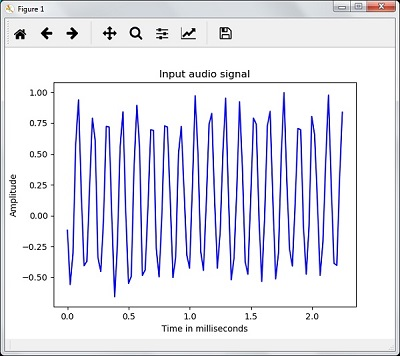```Signal shape: (132300,)
Signal Datatype: int16
Signal duration: 3.0 seconds```

### Characterizing the Audio Signal: Transforming to Frequency Domain

Characterizing an audio signal involves converting the time domain signal into frequency domain, and understanding its frequency components, by. This is an important step because it gives a lot of information about the signal. You can use a mathematical tool like Fourier Transform to perform this transformation.

### Example

The following example shows, step-by-step, how to characterize the signal, using Python, which is stored in a file. Note that here we are using Fourier Transform mathematical tool to convert it into frequency domain.

Import the necessary packages, as shown here −

```import numpy as np
import matplotlib.pyplot as plt
from scipy.io import wavfile```

Now, read the stored audio file. It will return two values: the sampling frequency and the the audio signal. Provide the path of the audio file where it is stored as shown in the command here −

```frequency_sampling, audio_signal = wavfile.read("/Users/admin/sample.wav")
```

In this step, we will display the parameters like sampling frequency of the audio signal, data type of signal and its duration, using the commands given below −

```print('\nSignal shape:', audio_signal.shape)
print('Signal Datatype:', audio_signal.dtype)
print('Signal duration:', round(audio_signal.shape /
float(frequency_sampling), 2), 'seconds')```

In this step, we need to normalize the signal, as shown in the following command −

```audio_signal = audio_signal / np.power(2, 15)
```

This step involves extracting the length and half length of the signal. Use the following commands for this purpose −

```length_signal = len(audio_signal)
half_length = np.ceil((length_signal + 1) / 2.0).astype(np.int)
```

Now, we need to apply mathematics tools for transforming into frequency domain. Here we are using the Fourier Transform.

`signal_frequency = np.fft.fft(audio_signal)`

Now, do the normalization of frequency domain signal and square it −

```signal_frequency = abs(signal_frequency[0:half_length]) / length_signal
signal_frequency **= 2```

Next, extract the length and half length of the frequency transformed signal −

`len_fts = len(signal_frequency)`

Note that the Fourier transformed signal must be adjusted for even as well as odd case.

```if length_signal % 2:
signal_frequency[1:len_fts] *= 2
else:
signal_frequency[1:len_fts-1] *= 2```

Now, extract the power in decibal(dB) −

`signal_power = 10 * np.log10(signal_frequency)`

Adjust the frequency in kHz for X-axis −

`x_axis = np.arange(0, len_half, 1) * (frequency_sampling / length_signal) / 1000.0`

Now, visualize the characterization of signal as follows −

```plt.figure()
plt.plot(x_axis, signal_power, color='black')
plt.xlabel('Frequency (kHz)')
plt.ylabel('Signal power (dB)')
plt.show()```

You can observe the output graph of the above code as shown in the image below −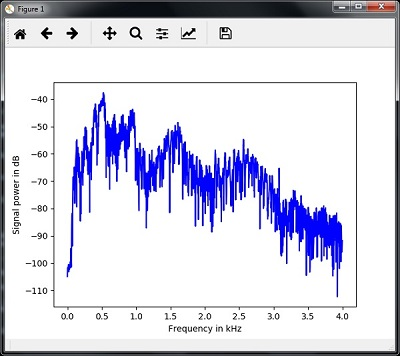### Generating Monotone Audio Signal

The two steps that you have seen till now are important to learn about signals. Now, this step will be useful if you want to generate the audio signal with some predefined parameters. Note that this step will save the audio signal in an output file.

### Example

In the following example, we are going to generate a monotone signal, using Python, which will be stored in a file. For this, you will have to take the following steps −

Import the necessary packages as shown −

```import numpy as np
import matplotlib.pyplot as plt
from scipy.io.wavfile import write```

Provide the file where the output file should be saved

```output_file = 'audio_signal_generated.wav'
```

Now, specify the parameters of your choice, as shown −

```duration = 4 # in seconds
frequency_sampling = 44100 # in Hz
frequency_tone = 784
min_val = -4 * np.pi
max_val = 4 * np.pi```

In this step, we can generate the audio signal, as shown −

```t = np.linspace(min_val, max_val, duration * frequency_sampling)
audio_signal = np.sin(2 * np.pi * tone_freq * t)```

Now, save the audio file in the output file −

```write(output_file, frequency_sampling, signal_scaled)
```

Extract the first 100 values for our graph, as shown −

```audio_signal = audio_signal[:100]
time_axis = 1000 * np.arange(0, len(signal), 1) / float(sampling_freq)```

Now, visualize the generated audio signal as follows −

```plt.plot(time_axis, signal, color='blue')
plt.xlabel('Time in milliseconds')
plt.ylabel('Amplitude')
plt.title('Generated audio signal')
plt.show()```

You can observe the plot as shown in the figure given here −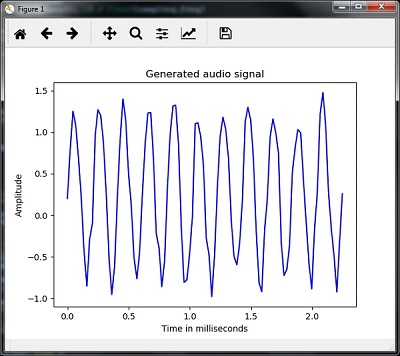### Feature Extraction from Speech

This is the most important step in building a speech recognizer because after converting the speech signal into the frequency domain, we must convert it into the usable form of feature vector. We can use different feature extraction techniques like MFCC, PLP, PLP-RASTA etc. for this purpose.

### Example

In the following example, we are going to extract the features from signal, step-by-step, using Python, by using MFCC technique.

Import the necessary packages, as shown here −

```import numpy as np
import matplotlib.pyplot as plt
from scipy.io import wavfile
from python_speech_features import mfcc, logfbank```

Now, read the stored audio file. It will return two values − the sampling frequency and the audio signal. Provide the path of the audio file where it is stored.

`frequency_sampling, audio_signal = wavfile.read("/Users/admin/audio_file.wav")`

Note that here we are taking first 15000 samples for analysis.

`audio_signal = audio_signal[:15000]`

Use the MFCC techniques and execute the following command to extract the MFCC features −

```features_mfcc = mfcc(audio_signal, frequency_sampling)
```

Now, print the MFCC parameters, as shown −

```print('\nMFCC:\nNumber of windows =', features_mfcc.shape)
print('Length of each feature =', features_mfcc.shape)```

Now, plot and visualize the MFCC features using the commands given below −

```features_mfcc = features_mfcc.T
plt.matshow(features_mfcc)
plt.title('MFCC')```

In this step, we work with the filter bank features as shown −

Extract the filter bank features −

`filterbank_features = logfbank(audio_signal, frequency_sampling)`

Now, print the filterbank parameters.

```print('\nFilter bank:\nNumber of windows =', filterbank_features.shape)
print('Length of each feature =', filterbank_features.shape)```

Now, plot and visualize the filterbank features.

```filterbank_features = filterbank_features.T
plt.matshow(filterbank_features)
plt.title('Filter bank')
plt.show()```

As a result of the steps above, you can observe the following outputs: Figure1 for MFCC and Figure2 for Filter Bank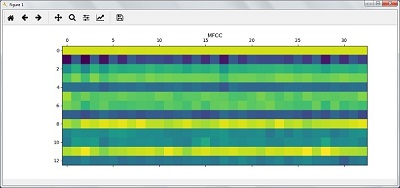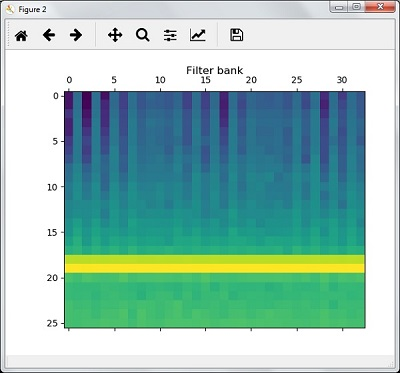### Recognition of Spoken Words

Speech recognition means that when humans are speaking, a machine understands it. Here we are using Google Speech API in Python to make it happen. We need to install the following packages for this −

• Pyaudio − It can be installed by using pip install Pyaudio command.
• SpeechRecognition − This package can be installed by using pip install SpeechRecognition.
• Google-Speech-API − It can be installed by using the command pip install google-api-python-client.

### Example

Observe the following example to understand about recognition of spoken words −

Import the necessary packages as shown −

`import speech_recognition as sr`

Create an object as shown below −

`recording = sr.Recognizer()`

Now, the Microphone() module will take the voice as input −

```with sr.Microphone() as source: recording.adjust_for_ambient_noise(source)
audio = recording.listen(source)```

Now google API would recognize the voice and gives the output.

```try:
except Exception as e:
print(e)
```

You can see the following output −

```Please Say Something:
You said:
```

For example, if you said tutorialspoint.com, then the system recognizes it correctly as follows −

`tutorialspoint.com`

[wpsbx_html_block id=1891]## Part 4: Artificial Intelligence on Natural Language Processing(NLP)

Natural Language Processing (NLP) refers to AI method of communicating with an intelligent systems using a natural language such as English. Processing of Natural Language is required when you want an intelligent system like robot to perform as per your instructions, when you want to hear decision from a dialogue based clinical expert system, etc. The field of NLP involves making computers to perform useful tasks with the natural languages humans use. The input and output of an NLP system can be −

• Speech
• Written Text

## Components of NLP

There are two components of NLP as given:

### Natural Language Understanding (NLU)

Understanding involves the following tasks −

• Mapping the given input in natural language into useful representations.
• Analyzing different aspects of the language.

### Natural Language Generation (NLG)

It is the process of producing meaningful phrases and sentences in the form of natural language from some internal representation.

It involves :

• Text planning − It includes retrieving the relevant content from knowledge base.
• Sentence planning − It includes choosing required words, forming meaningful phrases, setting tone of the sentence.
• Text Realization − It is mapping sentence plan into sentence structure.

The NLU is harder than NLG.

### Difficulties in NLU

NLP has an extremely rich form and structure. It is very ambiguous. There can be different levels of ambiguity −

• Lexical ambiguity − It is at very primitive level such as word-level.
• For example, treating the word “board” as noun or verb?
• Syntax Level ambiguity − A sentence can be parsed in different ways.
• For example, “He lifted the beetle with red cap.” − Did he use cap to lift the beetle or he lifted a beetle that had red cap?
• Referential ambiguity − Referring to something using pronouns. For example, Rima went to Gauri. She said, “I am tired.” − Exactly who is tired?
• One input can mean different meanings.
• Many inputs can mean the same thing.

### NLP Terminology

• Phonology − It is study of organizing sound systematically.
• Morphology − It is a study of construction of words from primitive meaningful units.
• Morpheme − It is primitive unit of meaning in a language.
• Syntax − It refers to arranging words to make a sentence. It also involves determining the structural role of words in the sentence and in phrases.
• Semantics − It is concerned with the meaning of words and how to combine words into meaningful phrases and sentences.
• Pragmatics − It deals with using and understanding sentences in different situations and how the interpretation of the sentence is affected.
• Discourse − It deals with how the immediately preceding sentence can affect the interpretation of the next sentence.
• World Knowledge − It includes the general knowledge about the world.

[wpsbx_html_block id=1891]

### Steps in NLP

There are general five(5) steps:

• Lexical Analysis − It involves identifying and analyzing the structure of words. Lexicon of a language means the collection of words and phrases in a language. Lexical analysis is dividing the whole chunk of txt into paragraphs, sentences,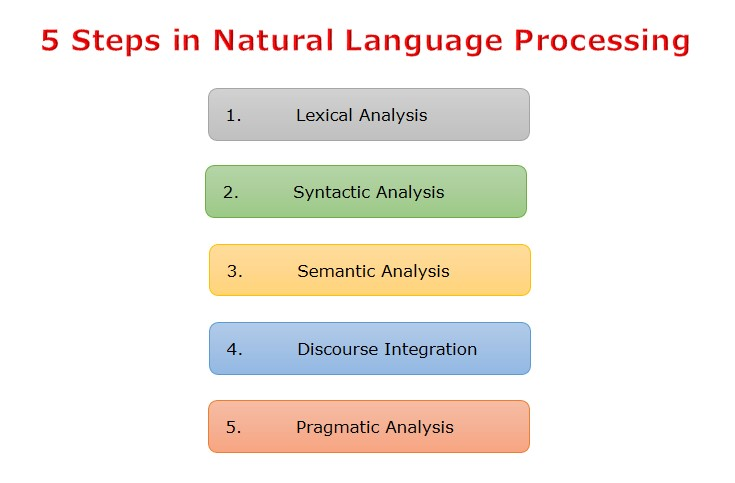and words.
• Syntactic Analysis (Parsing) − It involves analysis of words in the sentence for grammar and arranging word
• s in a manner that shows the relationship among the words. The sentence such as “The school goes to boy” is rejected by English syntactic analyzer.
• Semantic Analysis − It draws the exact meaning or the dictionary meaning from the text. The text
is checked for meaningfulness. It is done by mapping syntactic structures and objects in the task domain. The semantic analyzer disregards sentence such as “hot ice-cream”.
• Discourse Integration − The meaning of any sentence depends upon the meaning of the sentence just before it. In addition, it also brings about the meaning of immediately succeeding sentence.
• Pragmatic Analysis − During this, what was said is re-interpreted on what it actually meant. It involves deriving those aspects of language which require real world knowledge.

### Implementation Aspects of Syntactic Analysis

There are a number of algorithms researchers have developed for syntactic analysis, but we consider only the following simple methods:

• Context-Free Grammar
• Top-Down Parser

Let us see them in detail:

### Context-Free Grammar

It is the grammar that consists rules with a single symbol on the left-hand side of the rewrite rules. Let us create grammar to parse a sentence:

“The bird pecks the grains”

Articles (DET) − a | an | the

Nouns − bird | birds | grain | grains

Noun Phrase (NP) − Article + Noun | Article + Adjective + Noun

= DET N | DET ADJ N

Verbs − pecks | pecking | pecked

Verb Phrase (VP) − NP V | V NP

The parse tree breaks down the sentence into structured parts so that the computer can easily understand and process it. In order for the parsing algorithm to construct this parse tree, a set of rewrite rules, which describe what tree structures are legal, need to be constructed. These rules say that a certain symbol may be expanded in the tree by a sequence of other symbols. According to first order logic rule, if there are two strings Noun Phrase (NP) and Verb Phrase (VP), then the string combined by NP followed by VP is a sentence. The rewrite rules for the sentence are as follows −

S → NP VP

NP → DET N | DET ADJ N

VP → V NP

Lexocon −

DET → a | the

N → bird | birds | grain | grains

V → peck | pecks | pecking

The parse tree can be created as shown −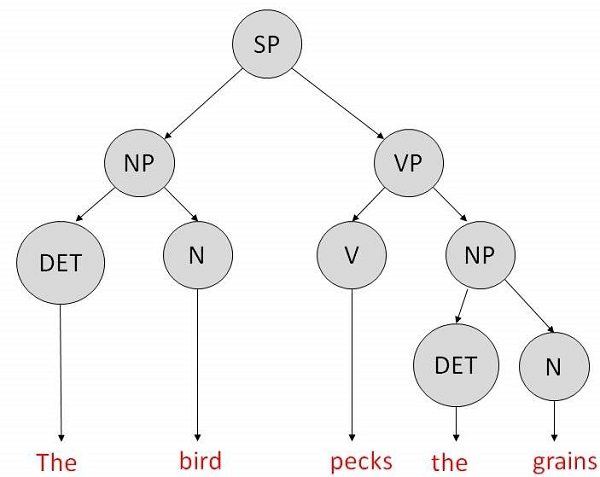Now consider the above rewrite rules. Since V can be replaced by both, “peck” or “pecks”, sentences such as “The bird peck the grains” can be wrongly permitted. i. e. the subject-verb agreement error is approved as correct.

Merit:The simplest style of grammar, therefore widely used one.

Demerits:

• They are not highly precise. For example, “The grains peck the bird”, is a syntactically correct according to parser, but even if it makes no sense, parser takes it as a correct sentence.
• To bring out high precision, multiple sets of grammar need to be prepared. It may require a completely different sets of rules for parsing singular and plural variations, passive sentences, etc., which can lead to creation of huge set of rules that are unmanageable.

### Top-Down Parser

Here, the parser starts with the S symbol and attempts to rewrite it into a sequence of terminal symbols that matches the classes of the words in the input sentence until it consists entirely of terminal symbols. These are then checked with the input sentence to see if it matched. If not, the process is started over again with a different set of rules. This is repeated until a specific rule is found which describes the structure of the sentence.

Merit: It is simple to implement.

Demerits:

• It is inefficient, as the search process has to be repeated if an error occurs.
• Slow speed of working.